We also don’t want to dig too deep into the mathematical rabbit hole

$$y^2=x^3+ax+b$$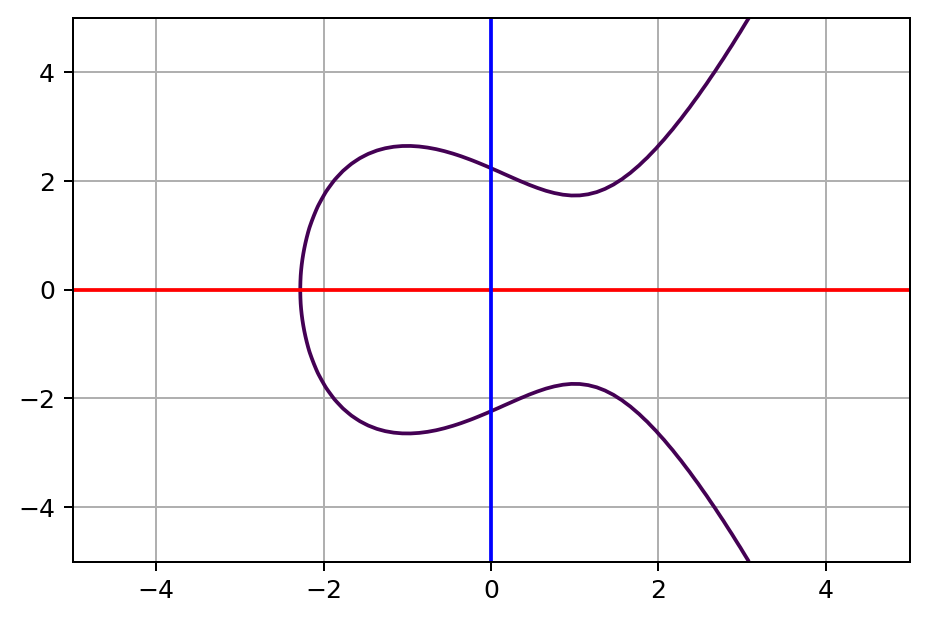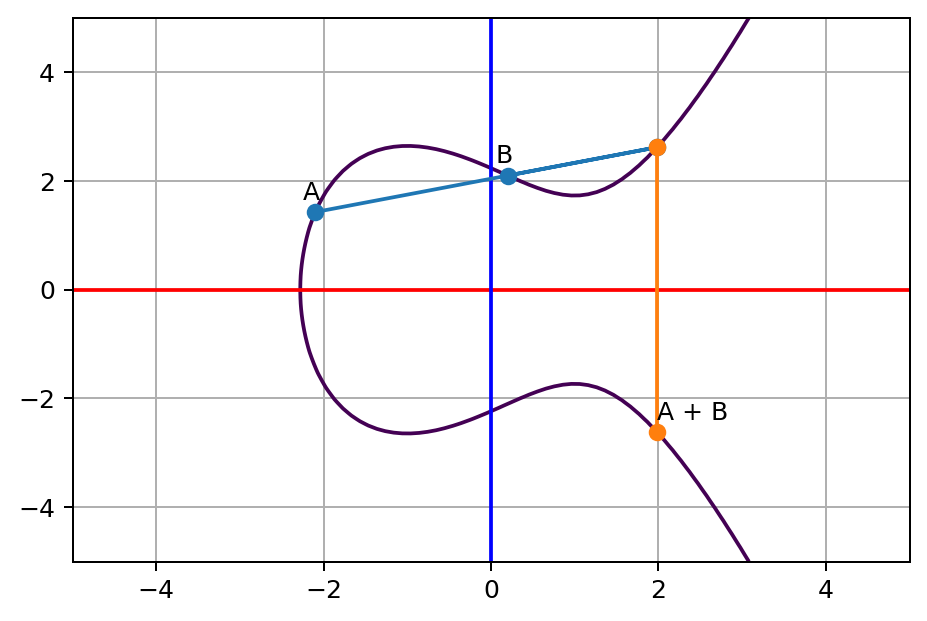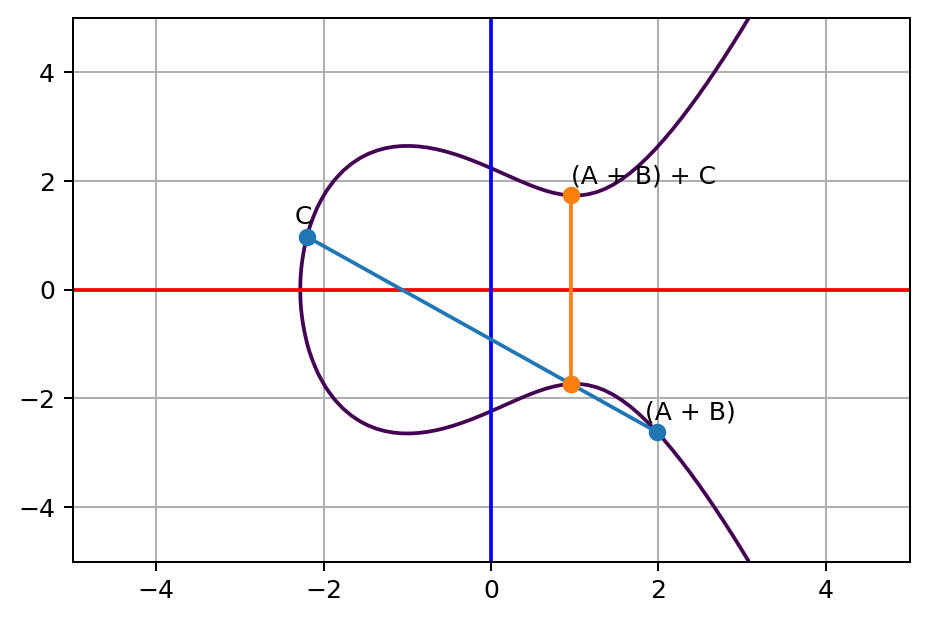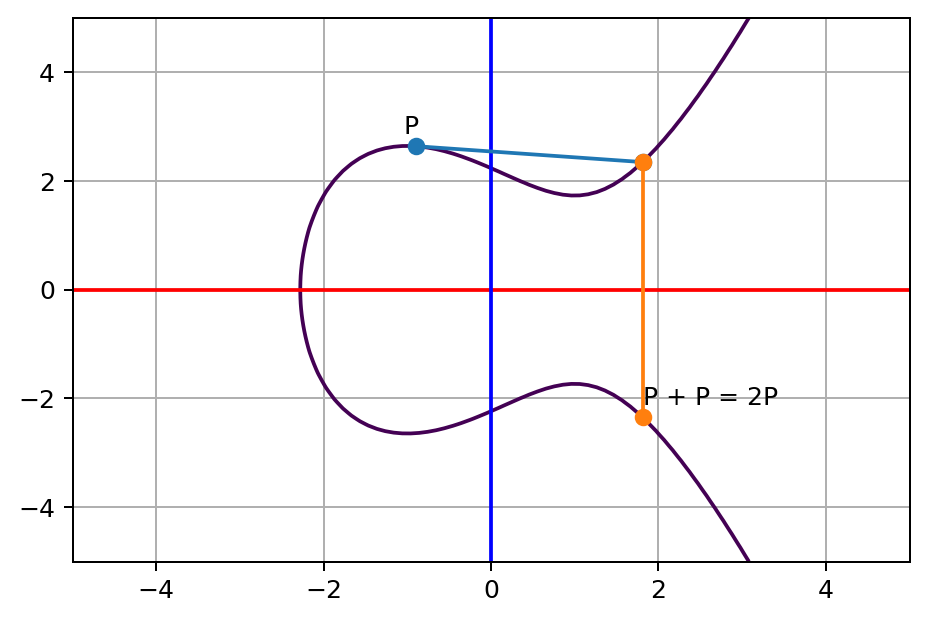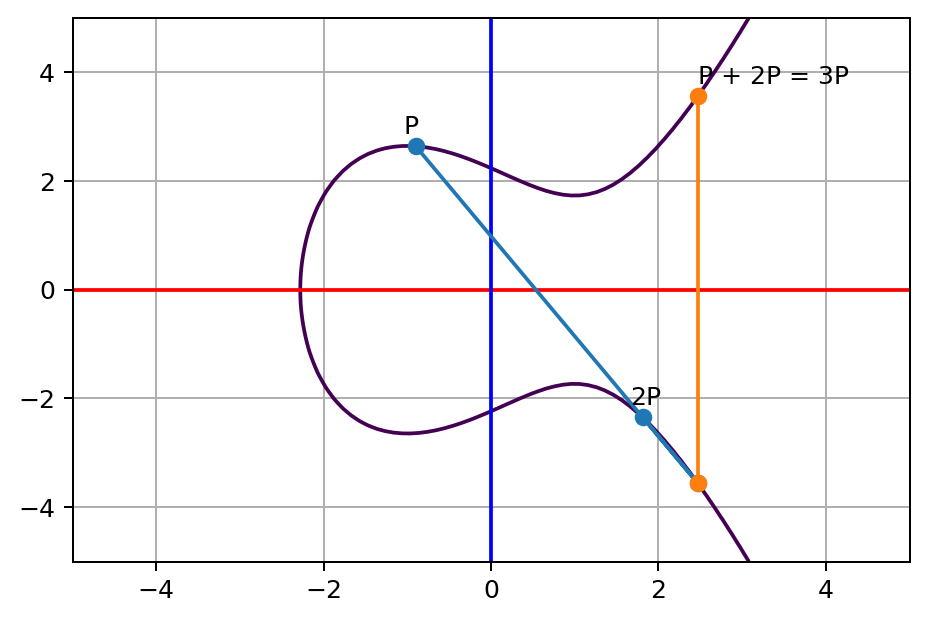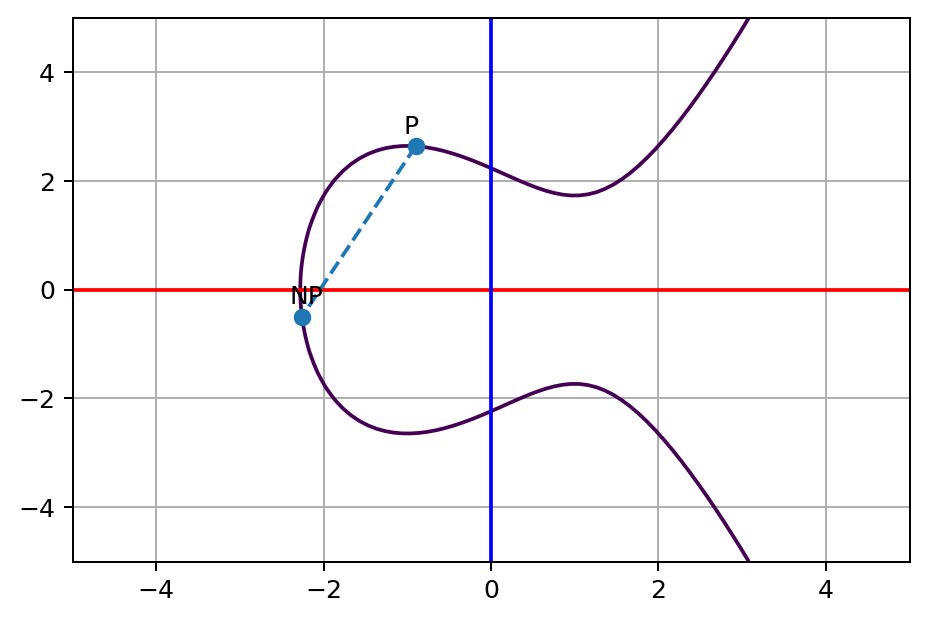$$a+(b+c)=(a+b)+c$$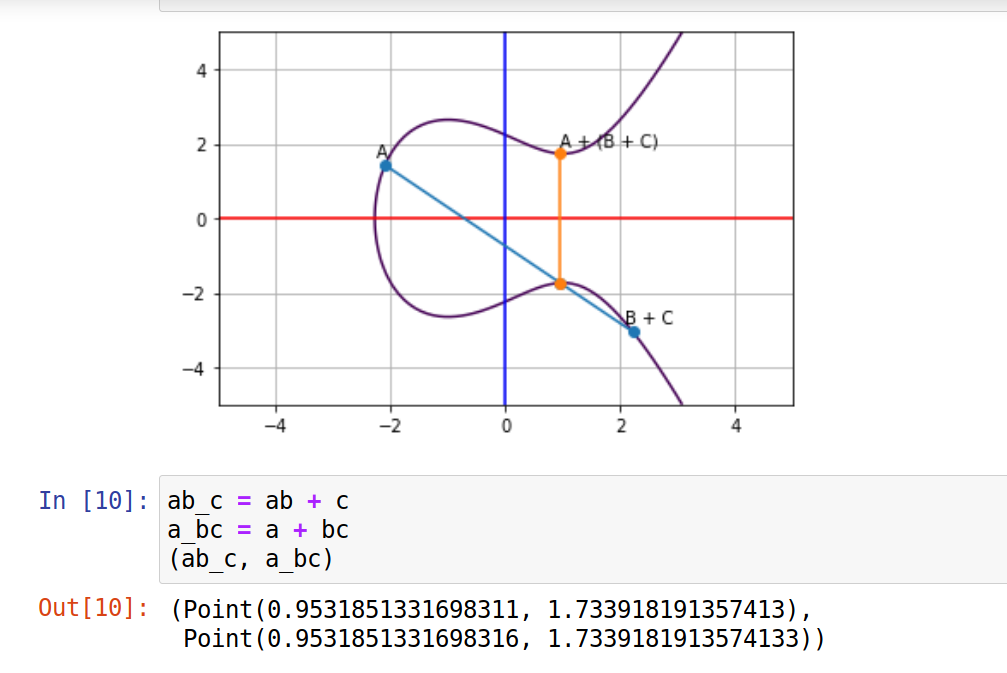$$P+3P=4P=2P+2P$$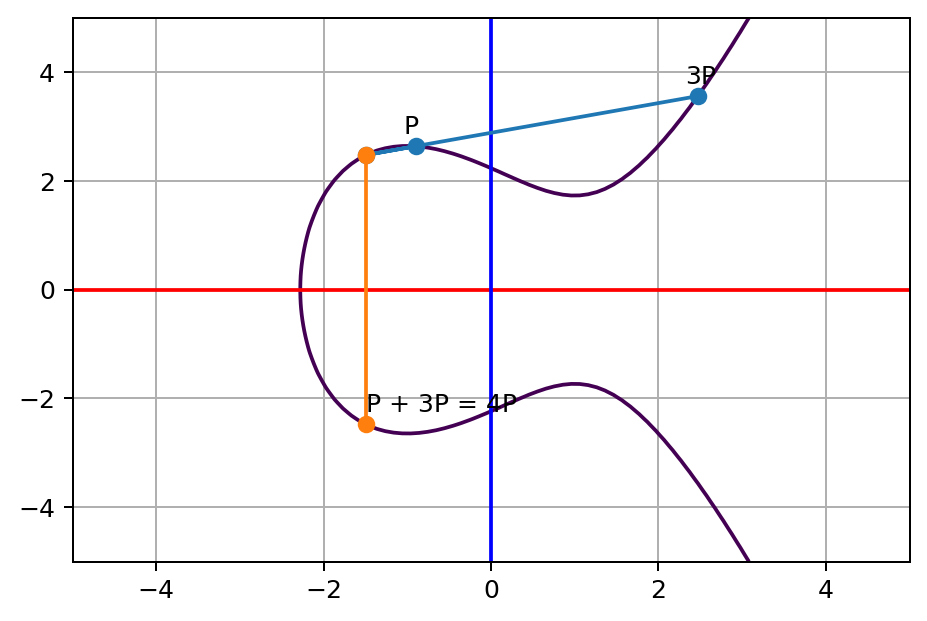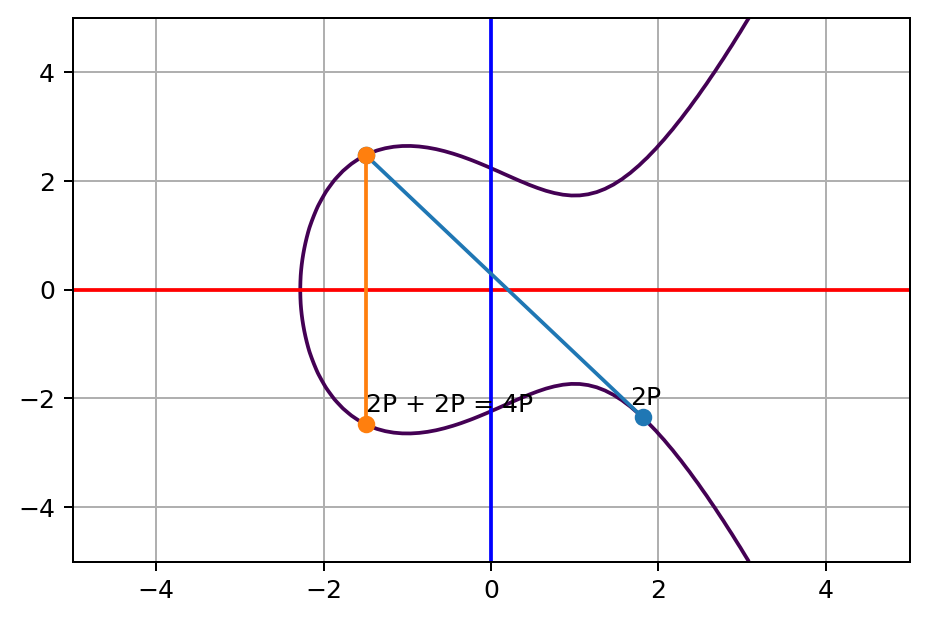$$11100011$$

$$2^7P+2^6P+2^5P+2^1P+2^0P$$

$$128P+64P+32P+2P+P$$

$$a^x+a^y=a^{x+y}$$

$$3^4 = 3\times 3\times 3\times 3=81$$

$$4=log_381$$

Namely, find out what’s the N value from NP and P point given if N is big enough. This is called Elliptic Curve Discrete Logarithm Problem.

$$SK=NP\times M=MP \times N$$

Elliptic Curve Cryptography Explained

How To Set Up Jupyter Notebook with Python 3 on Ubuntu 18.04 | DigitalOcean